Name:    Chapter 7 Post Test

Multiple Choice
Identify the choice that best completes the statement or answers the question.

1.

Solve the system by the method of substitution.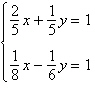a. (4, 3) b. (4, –3) c. (3, 4) d. (–3, 4) e. (–4, 3)

2.

Solve the system by the method of substitution.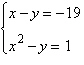a. (–4, –23), (5, –14) b. no real solution c. (–4, –25) d. (–4, –25), (5, –16) e. (–4, 15), (5, 24)

3.

Use a graphing utility to solve the system of equations. Find the solution accurate to two decimal places.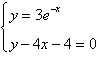a.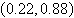b.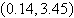c.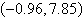d.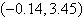e. no real solution

4.

Solve the system by the method of substitution.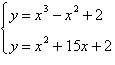a. (5, 102), (3, 56), (1, 18) b. (5, 102), (–1, –12) c. (–3, –34), (1, 18) d. (5, 102), (–3, –34), (0, 2) e. no real solution

5.

Find the sales necessary to break even (R – C = 0) for the cost C of producing x units and the revenue R obtained by selling x units. (Round to the nearest whole unit.)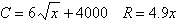a. 782 units or 852 units b. no real solution c. 852 units d. 782 units e. 831 units

6.

Solve the system by the method of elimination.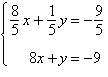a.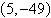b.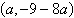(dependent) c.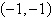d.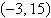e. inconsistent

7.

Solve the system by the method of elimination.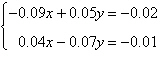a. inconsistent b.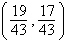c.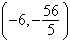d.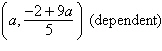e.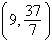8.

Solve the system by the method of elimination.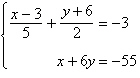a.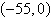b.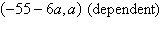c. inconsistent d.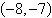e.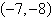9.

Find the least squares regression line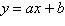for the points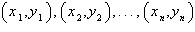by solving the system for a and b.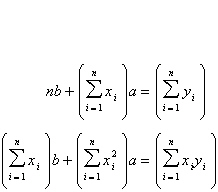Points: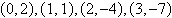space
 a. y = –3.47x –4.21 b. y = 3.93x –4.21 c. y = 2.80x –3.20 d. y = –2.66x +2.80 e. y = –3.20x +2.80

10.

Solve the system of linear equations.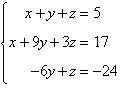a.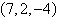b.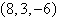c.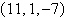d.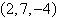e.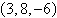11.

Write the form of the partial fraction decomposition of the rational expression. Do not solve for the constants.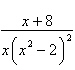a.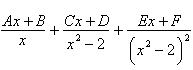b.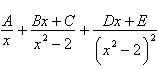c.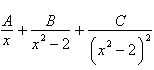d.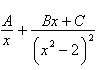e.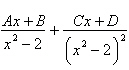12.

Write the partial fraction decomposition of the rational expression.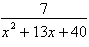a.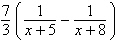b.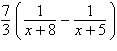c.d.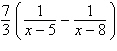e.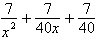13.

Write the partial fraction decomposition of the rational expression.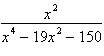a.b.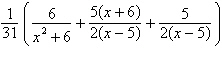c.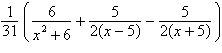d.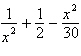e.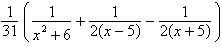14.

Write the partial fraction decomposition of the improper rational expression.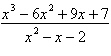a.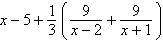b.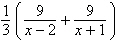c.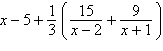d.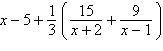e.15.

Use a graphing utility to graph the inequality. Shade the region representing the solution.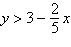a.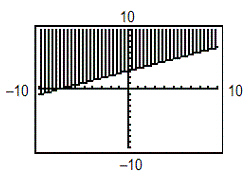d.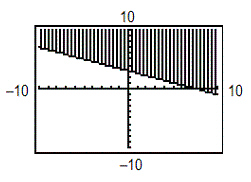b.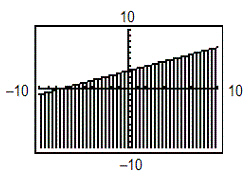e.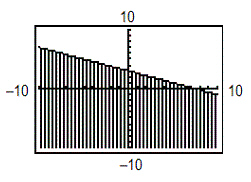c.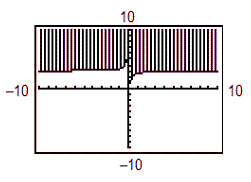16.

Write an inequality for the shaded region shown in the figure.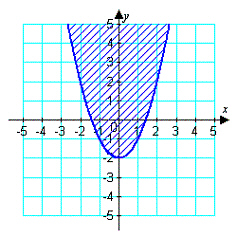a.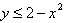b.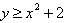c.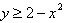d.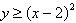e.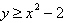17.

Sketch the graph and label the vertices of the solution set of the system of inequalities. Shade the solution set.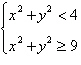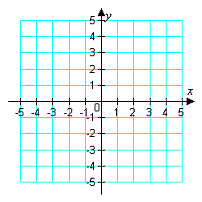a.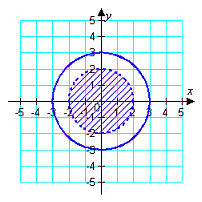d.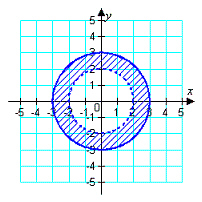b.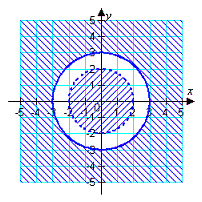e.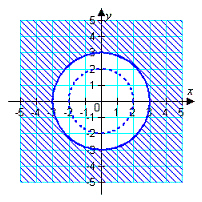c.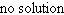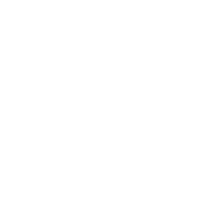18.

Derive a set of inequalities to describe the region.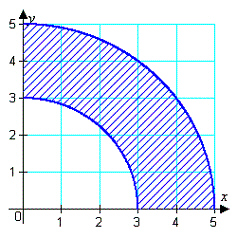a.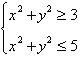b.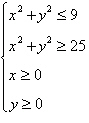c.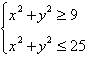d.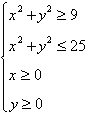e.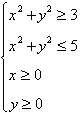19.

Find the minimum and maximum values of the objective function and where they occur, subject to the indicated constraints.
 Objective function:Constraints: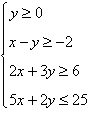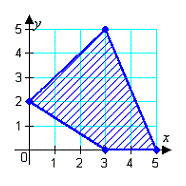a.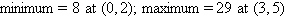b.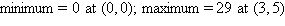c.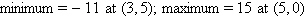d.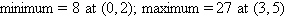e.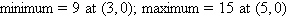20.

An investor has \$150,000 to invest in two types of investments. Type A pays 5% annually and type B pays 6% annually. To have a well-balanced portfolio, the investor imposes the following conditions. At least one-third of the total portfolio is to be allocated to type A investments and at least one-third of the portfolio is to be allocated to type B investments. What is the optimal amount that should be invested in each investment?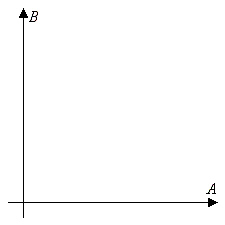a. \$150,000 in type A (5%), \$0 in type B (6%) b. \$50,000 in type A (5%), \$100,000 in type B (6%) c. \$100,000 in type A (5%), \$50,000 in type B (6%) d. \$0 in type A (5%), \$150,000 in type B (6%) e. \$60,000 in type A (5%), \$90,000 in type B (6%)